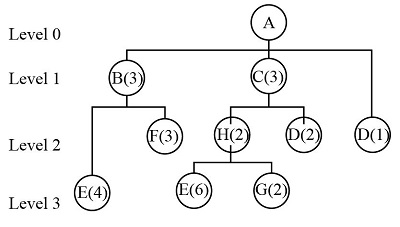# Product A consists of two units of Subassembly B, three units of C, and one unit of D. B is...

## Question:

Product A consists of two units of Subassembly B, three units of C, and one unit of D. B is composed of four units of E and three units of F. C is made of two units of H and two units of D. H is made of six units of E and two units of G. To produce 100 units of A, determine the numbers of units of B, C, D, E, F, G, and H required using the low-level coded product structure tree. Level 0 100 units of A Level 1 units of B units of C Level 2 units of F units of H units of D Level 3 units of E units of G

## Decision Tree:

Decision tree refers to the technique used by the business for a better understanding of the planning process. It refers to a hierarchy model that provides information from high to lower level or lower to higher level.

With the given information, the low-level coded product structure tree would be:According to the tree, the required amount of all the products to produce 100 units of product A would be:

Product B:

{eq}\begin{array}{l} {\rm{ = 3 \times units of product A}}\\ {\rm{ = 3}} \times {\rm{100}}\\ {\rm{ = 300}} \end{array} {/eq}

Product C:

{eq}\begin{array}{l} {\rm{ = 3 \times units of product A}}\\ {\rm{ = 3}} \times {\rm{100}}\\ {\rm{ = 300}} \end{array} {/eq}

Product F:

{eq}\begin{array}{l} {\rm{ = 3 \times units of product B}}\\ {\rm{ = 3}} \times {\rm{300}}\\ {\rm{ = 900}} \end{array} {/eq}

Product H:

{eq}\begin{array}{l} {\rm{ = 2 \times units of product C}}\\ {\rm{ = 2}} \times 3{\rm{00}}\\ {\rm{ = 600}} \end{array} {/eq}

Product D:

{eq}\begin{array}{l} {\rm{ = }}\left( {{\rm{2 \times units of product C}}} \right){\rm{ + }}\left( {{\rm{1 \times units of product A}}} \right)\\ {\rm{ = }}\left( {{\rm{2}} \times 3{\rm{00}}} \right) + \left( {1 \times 100} \right)\\ {\rm{ = 600 + 100}}\\ {\rm{ = 700}} \end{array} {/eq}

Product E_:

{eq}\begin{array}{l} {\rm{ = }}\left( {{\rm{4 \times units of product B}}} \right){\rm{ + }}\left( {{\rm{6 \times units of product H}}} \right)\\ {\rm{ = }}\left( {4 \times 3{\rm{00}}} \right) + \left( {6 \times 600} \right)\\ {\rm{ = 1200 + 3600}}\\ {\rm{ = 4800}} \end{array} {/eq}

Product G:

{eq}\begin{array}{l} {\rm{ = 2 \times units of product H}}\\ {\rm{ = 2}} \times 6{\rm{00}}\\ {\rm{ = 1200}} \end{array} {/eq}

Hence,

 Level Units Product 0 100 A 1 300 B

 300 C 2 900 F

600 H

 700 D 3 4800 E

1200 GWhat Is a Decision Tree? - Examples, Advantages & Role in Management

from

Chapter 2 / Lesson 12
61K

Clearly identifying all possible solutions for a given decision is an important part of successful management. In this lesson, you will learn how to use a decision tree to identify and select possible courses of action.